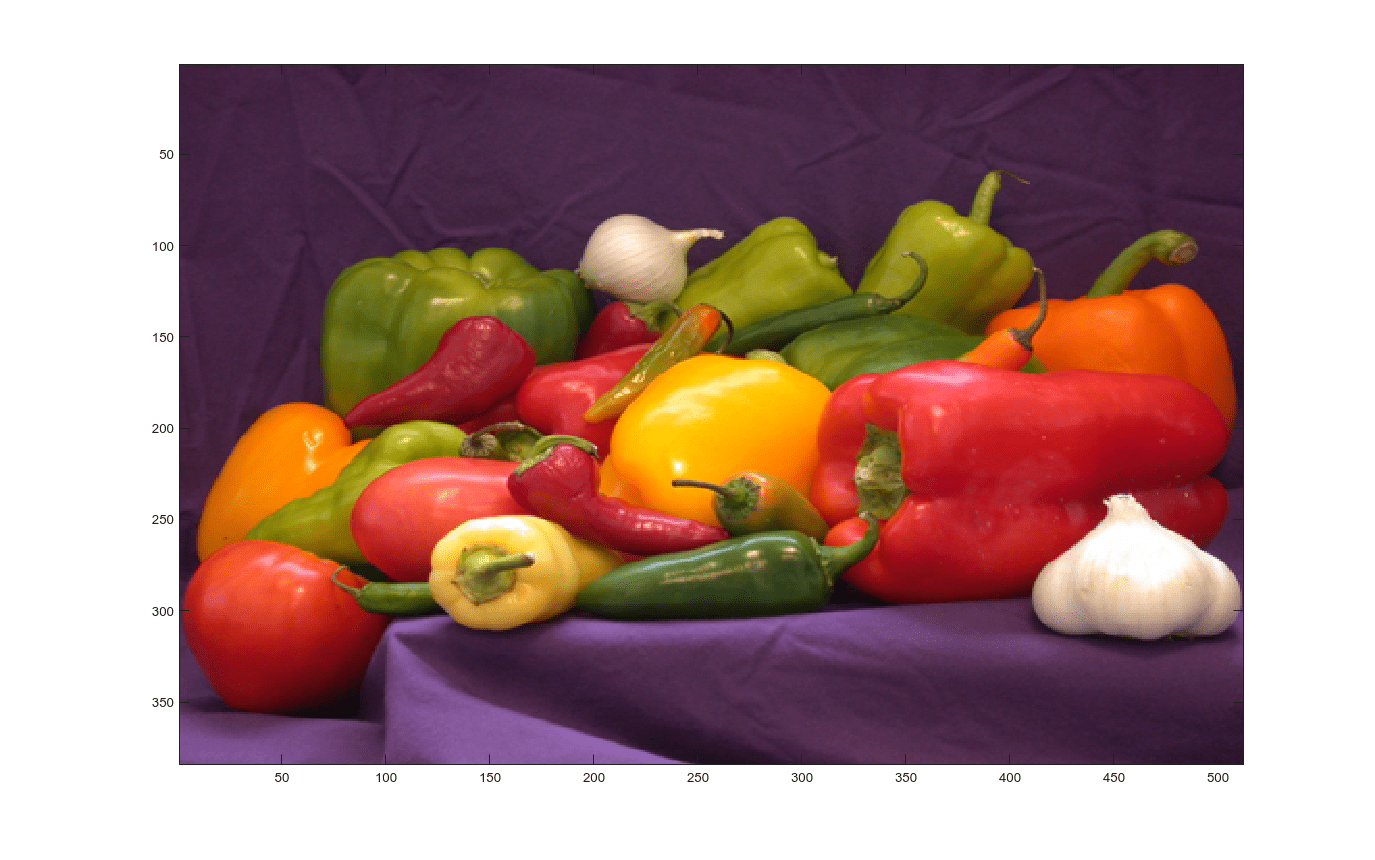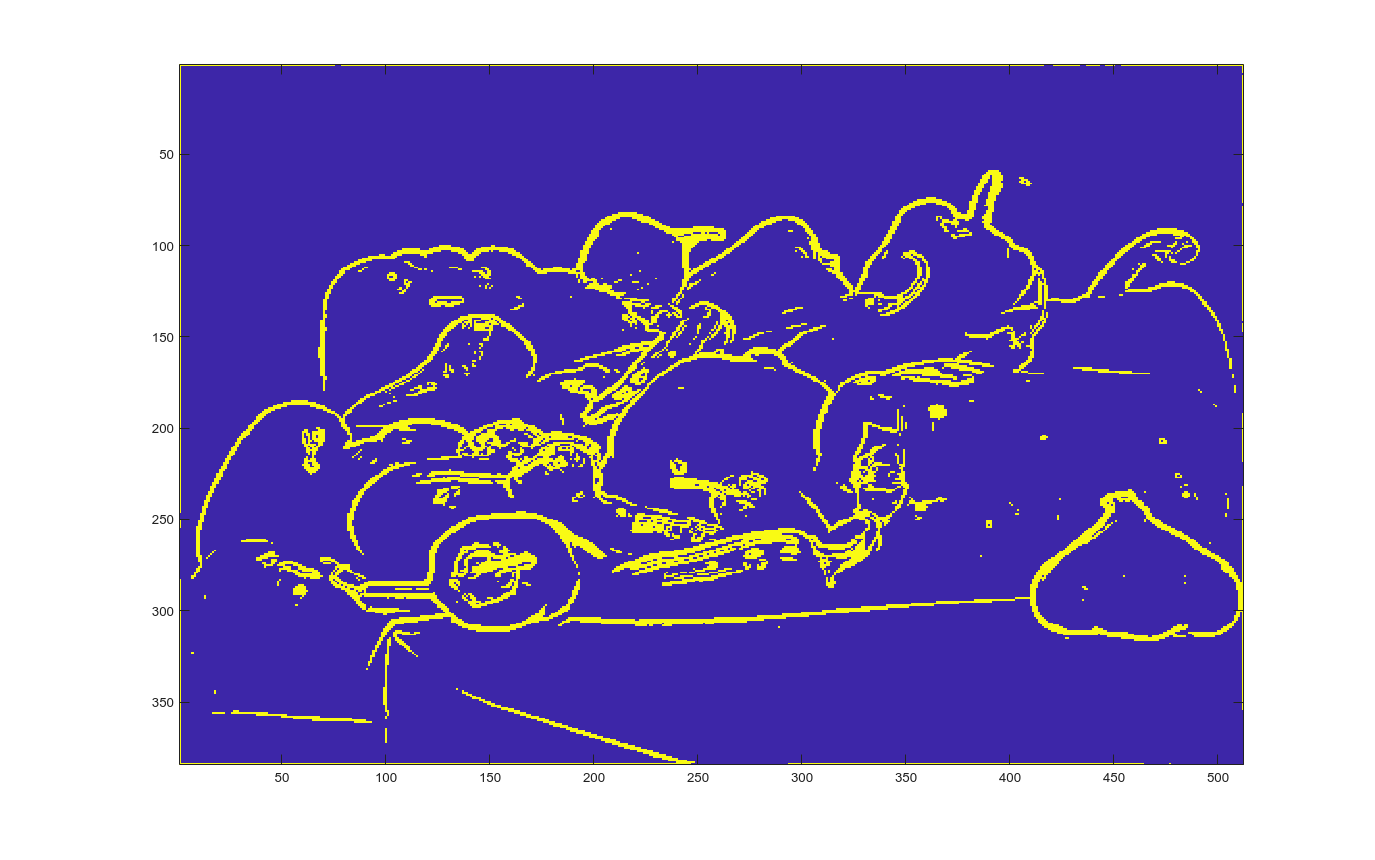# Edge Detection with Sobel Method in Half-Precision

This example demonstrates edge detection in an image with a CUDA® MEX function generated from a MATLAB® function. The edge detection algorithm is implemented with half-precision data type.

### Third-Party Prerequisites

Required

This example generates CUDA MEX and has the following third-party requirements.

• CUDA enabled NVIDIA® GPU with a minimum compute capability of 5.3 and compatible driver.

Optional

For non-MEX builds such as static, dynamic libraries or executables, this example has the following additional requirements.

### Verify GPU Environment

To verify that the compilers and libraries necessary for running this example are set up correctly, use the `coder.checkGpuInstall` function.

```envCfg = coder.gpuEnvConfig('host'); envCfg.BasicCodegen = 1; envCfg.Quiet = 1; coder.checkGpuInstall(envCfg); ```

### Sobel Edge Detection Algorithm

In the Sobel edge detection algorithm (sobelEdgeDetectionAlg.m) , a 2-D spatial gradient operation is performed on a gray scale image. This operation emphasizes the high spatial frequency regions that correspond to the edges in the image.

```type sobelEdgeDetectionAlg ```
```function edgeImg = sobelEdgeDetectionAlg(img,thresh) %#codegen %sobelEdgeDetection Example MATLAB function for edge detection. % Copyright 2018 The MathWorks, Inc. kern = half([1 2 1; 0 0 0; -1 -2 -1]); % Finding horizontal and vertical gradients. h = conv2(img(:,:,2),kern,'same'); v = conv2(img(:,:,2),kern','same'); % Finding magnitude of the gradients. e = sqrt(h.*h + v.*v); % Threshold the edges edgeImg = uint8((e > thresh) * 240); end ```

The Sobel edge algorithm computes the horizontal gradient `resX` and the vertical gradient `resY` of the input image by using two orthogonal filter kernels `maskX` and `maskY`. After the filtering operation, the algorithm computes the gradient magnitude and applies a threshold to find the regions of the images that are considered to be edges.

### Read Images and Pack Data Into RGBA Packed Column Major Order

Use the standard `imread` command to read the images. `imread` represents the RGB channels of an images with integers, one for each pixel. The integers range from 0 to 255. Simply casting inputs to half type might result in overflow during convolutions. In this case, we can scale the images to values between 0 and 1.

```im = imread('peppers.png'); figure(); image(im); imPacked = half(im)/255; thresh = half(100)/255; ```### Generate CUDA MEX for the Function

To generate CUDA MEX for the `sobelEdgeDetectionAlg` function, create a GPU code configuration object and run the `codegen` command. To generate and execute code with half-precision data types, CUDA compute capability of 5.3 or higher is required. Set the `ComputeCapability` property of the code configuration object to `'5.3'`. For half-precision, the memory allocation (malloc) mode for generating CUDA code must be set to 'Discrete'.

```cfg = coder.gpuConfig('mex'); cfg.GpuConfig.ComputeCapability = '5.3'; cfg.GpuConfig.MallocMode = 'Discrete'; codegen -config cfg -args {imPacked,thresh} sobelEdgeDetectionAlg; ```

### Run the MEX Function

After you generate a MEX function, you can verify that it has the same functionality as the original MATLAB entry-point function. Run the generated `sobelEdgeDetectionAlg_mex` and plot the results.

```out_disp = sobelEdgeDetectionAlg_mex(imPacked,thresh); imagesc(out_disp); ```### Clear MEX Memory.

Clear the static network object that was loaded in memory.

```clear mex; ```